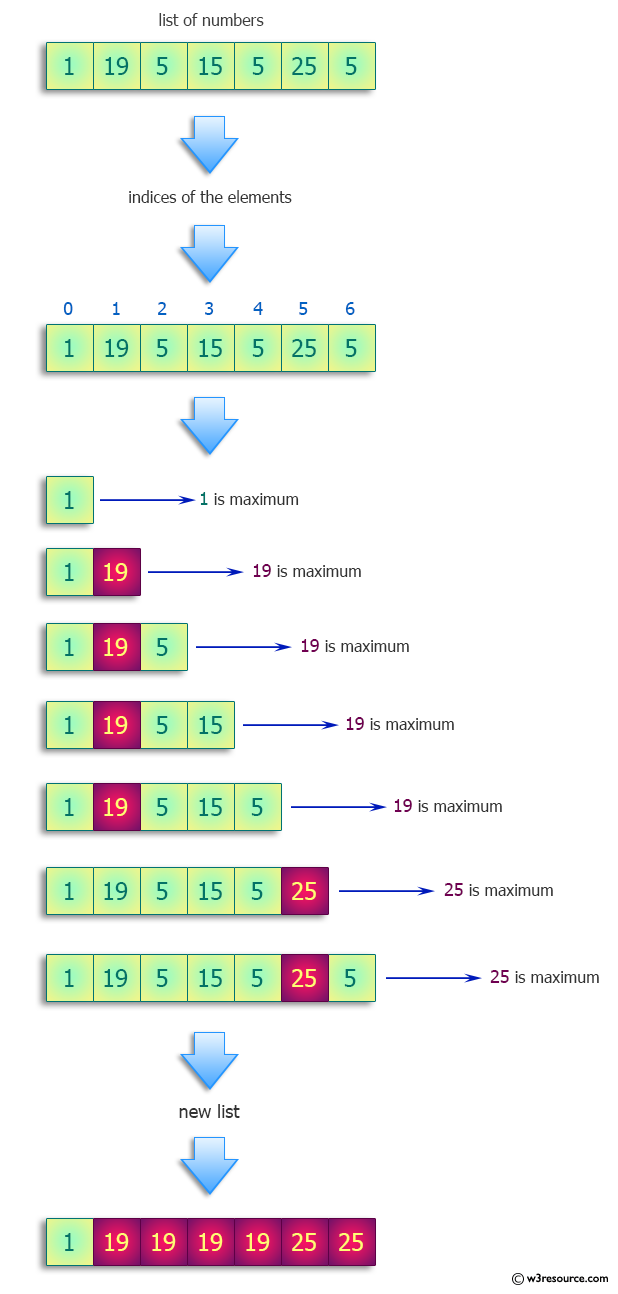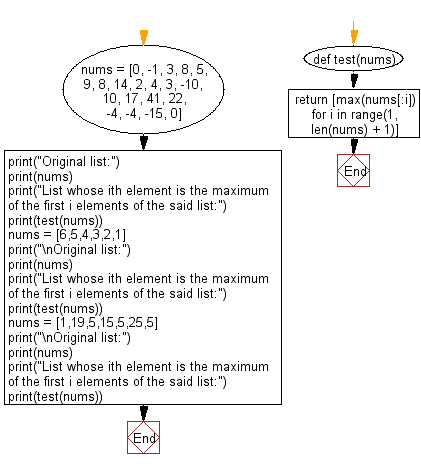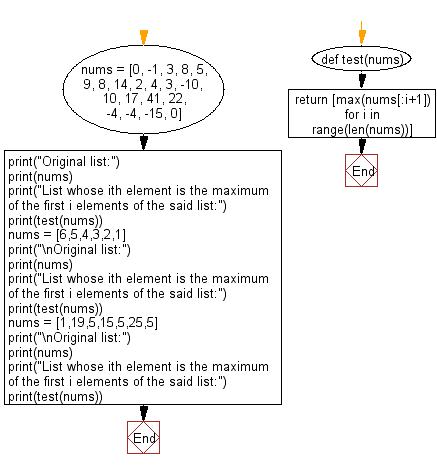﻿ Python: Create a list whose ith element is the maximum of the first i elements of the input list - w3resource# Python: Create a list whose ith element is the maximum of the first i elements of the input list

## Python Programming Puzzles: Exercise-24 with Solution

Write a Python program to create a list whose ith element is the maximum of the first i elements from a input list.

```Input:
[0, -1, 3, 8, 5, 9, 8, 14, 2, 4, 3, -10, 10, 17, 41, 22, -4, -4, -15, 0]
Output:
[0, 0, 3, 8, 8, 9, 9, 14, 14, 14, 14, 14, 14, 17, 41, 41, 41, 41, 41, 41]

Input:
[6, 5, 4, 3, 2, 1]
Output:
[6, 6, 6, 6, 6, 6]

Input:
[1, 19, 5, 15, 5, 25, 5]
Output:
[1, 19, 19, 19, 19, 25, 25]
```

Pictorial Presentation:Sample Solution-1:

Python Code:

``````#License: https://bit.ly/3oLErEI

def test(nums):
return [max(nums[:i]) for i in range(1, len(nums) + 1)]
nums = [0, -1, 3, 8, 5, 9, 8, 14, 2, 4, 3, -10, 10, 17, 41, 22, -4, -4, -15, 0]
print("Original list:")
print(nums)
print("List whose ith element is the maximum of the first i elements of the said list:")
print(test(nums))
nums = [6,5,4,3,2,1]
print("\nOriginal list:")
print(nums)
print("List whose ith element is the maximum of the first i elements of the said list:")
print(test(nums))
nums = [1,19,5,15,5,25,5]
print("\nOriginal list:")
print(nums)
print("List whose ith element is the maximum of the first i elements of the said list:")
print(test(nums))
``````

Sample Output:

```Original list:
[0, -1, 3, 8, 5, 9, 8, 14, 2, 4, 3, -10, 10, 17, 41, 22, -4, -4, -15, 0]
List whose ith element is the maximum of the first i elements of the said list:
[0, 0, 3, 8, 8, 9, 9, 14, 14, 14, 14, 14, 14, 17, 41, 41, 41, 41, 41, 41]

Original list:
[6, 5, 4, 3, 2, 1]
List whose ith element is the maximum of the first i elements of the said list:
[6, 6, 6, 6, 6, 6]

Original list:
[1, 19, 5, 15, 5, 25, 5]
List whose ith element is the maximum of the first i elements of the said list:
[1, 19, 19, 19, 19, 25, 25]
```

Flowchart:## Visualize Python code execution:

The following tool visualize what the computer is doing step-by-step as it executes the said program:

Sample Solution-2:

Python Code:

``````#License: https://bit.ly/3oLErEI

def test(nums):
return [max(nums[:i+1]) for i in range(len(nums))]

nums = [0, -1, 3, 8, 5, 9, 8, 14, 2, 4, 3, -10, 10, 17, 41, 22, -4, -4, -15, 0]
print("Original list:")
print(nums)
print("List whose ith element is the maximum of the first i elements of the said list:")
print(test(nums))
nums = [6,5,4,3,2,1]
print("\nOriginal list:")
print(nums)
print("List whose ith element is the maximum of the first i elements of the said list:")
print(test(nums))
nums = [1,19,5,15,5,25,5]
print("\nOriginal list:")
print(nums)
print("List whose ith element is the maximum of the first i elements of the said list:")
print(test(nums))
``````

Sample Output:

```Original list:
[0, -1, 3, 8, 5, 9, 8, 14, 2, 4, 3, -10, 10, 17, 41, 22, -4, -4, -15, 0]
List whose ith element is the maximum of the first i elements of the said list:
[0, 0, 3, 8, 8, 9, 9, 14, 14, 14, 14, 14, 14, 17, 41, 41, 41, 41, 41, 41]

Original list:
[6, 5, 4, 3, 2, 1]
List whose ith element is the maximum of the first i elements of the said list:
[6, 6, 6, 6, 6, 6]

Original list:
[1, 19, 5, 15, 5, 25, 5]
List whose ith element is the maximum of the first i elements of the said list:
[1, 19, 19, 19, 19, 25, 25]
```

Flowchart:## Visualize Python code execution:

The following tool visualize what the computer is doing step-by-step as it executes the said program:

Python Code Editor :

Have another way to solve this solution? Contribute your code (and comments) through Disqus.

What is the difficulty level of this exercise?

Test your Programming skills with w3resource's quiz.

﻿

## Python: Tips of the Day

Clamps num within the inclusive range specified by the boundary values x and y:

Example:

```def tips_clamp_num(num,x,y):
return max(min(num, max(x, y)), min(x, y))
print(tips_clamp_num(2, 4, 6))
print(tips_clamp_num(1, -1, -6))
```

Output:

```4
-1
```